Publicité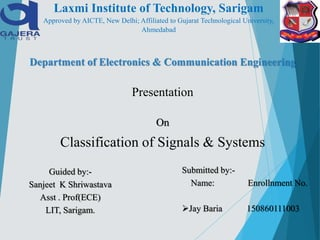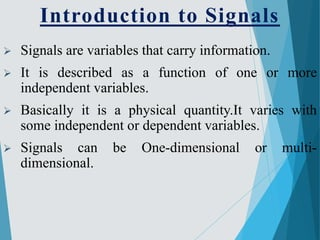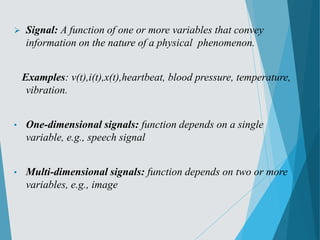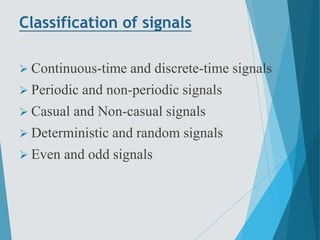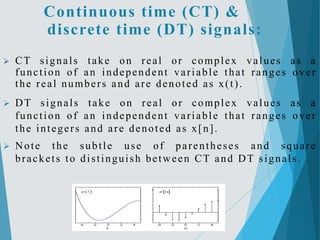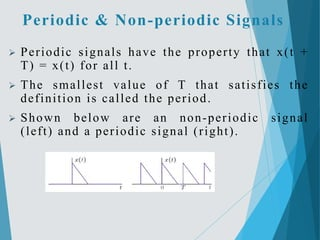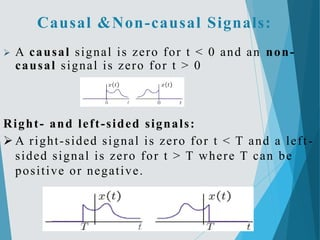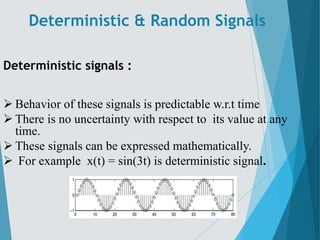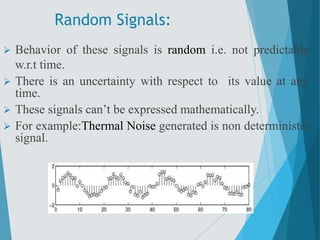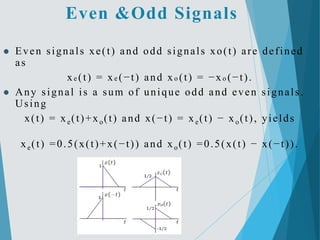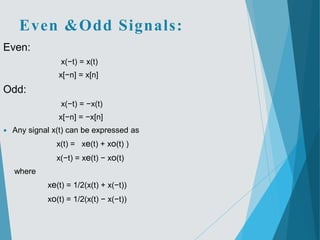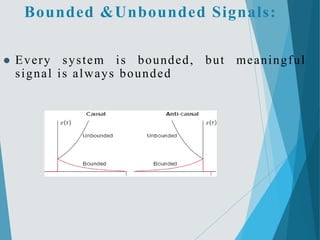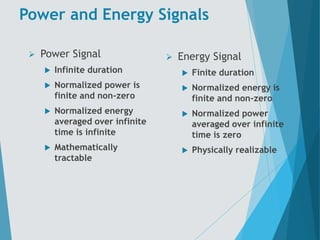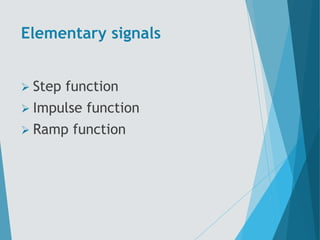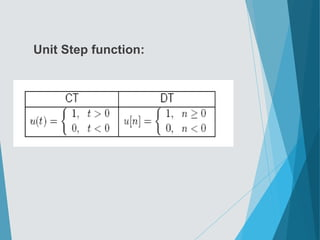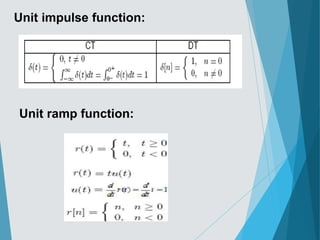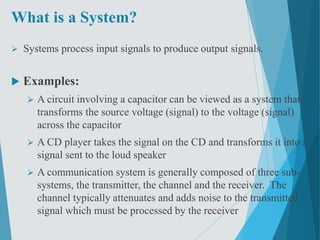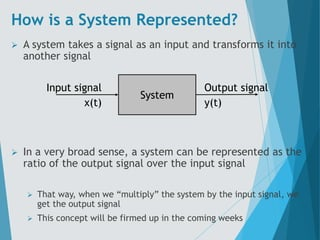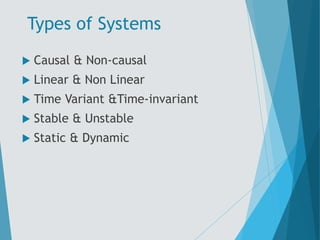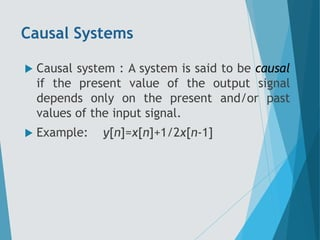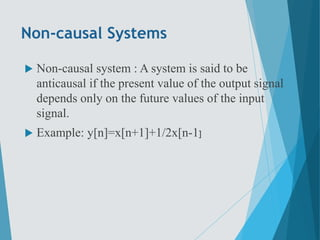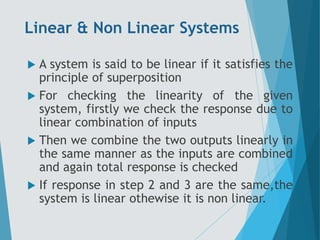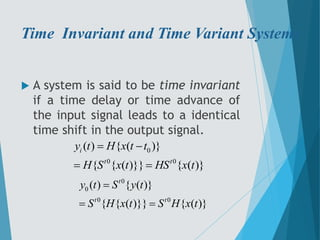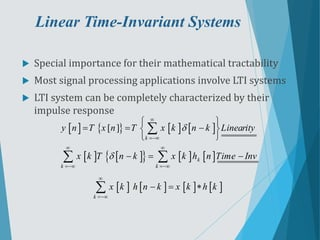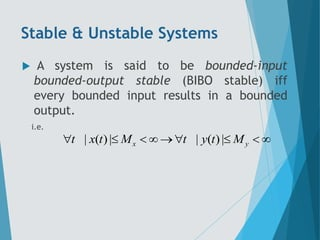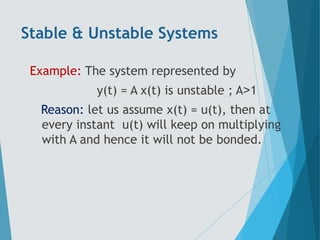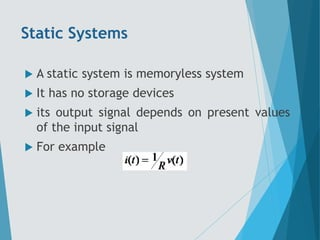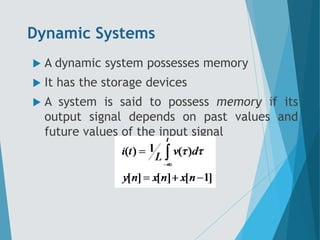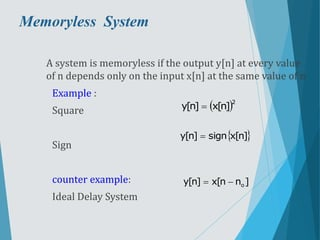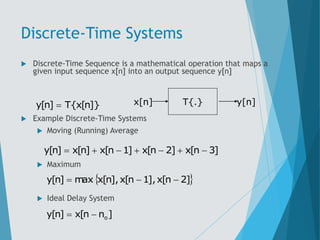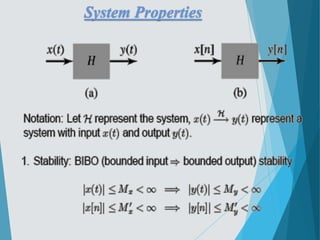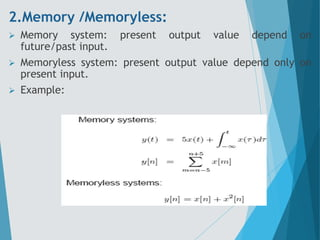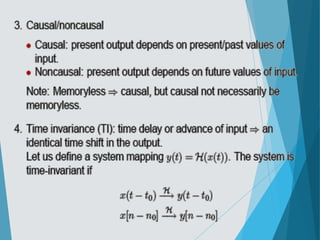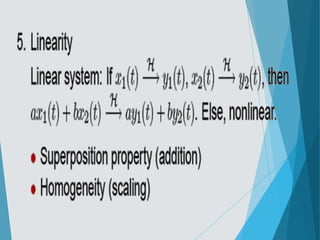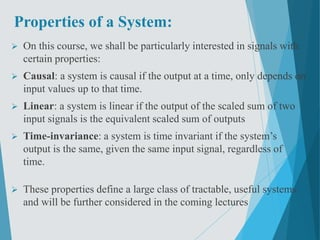1 sur 36
Publicité

### ssppt-170414031953.pdf

1. Department of Electronics & Communication Engineering Presentation On Classification of Signals & Systems Guided by:- Sanjeet K Shriwastava Asst . Prof(ECE) LIT, Sarigam. Submitted by:- Name: Enrollnment No. Jay Baria 150860111003 Laxmi Institute of Technology, Sarigam Approved by AICTE, New Delhi; Affiliated to Gujarat Technological University, Ahmedabad
2.  Signals are variables that carry information.  It is described as a function of one or more independent variables.  Basically it is a physical quantity.It varies with some independent or dependent variables.  Signals can be One-dimensional or multi- dimensional. Introduction to Signals
3.  Signal: A function of one or more variables that convey information on the nature of a physical phenomenon. Examples: v(t),i(t),x(t),heartbeat, blood pressure, temperature, vibration. • One-dimensional signals: function depends on a single variable, e.g., speech signal • Multi-dimensional signals: function depends on two or more variables, e.g., image
4. Classification of signals  Continuous-time and discrete-time signals  Periodic and non-periodic signals  Casual and Non-casual signals  Deterministic and random signals  Even and odd signals
5. Continuous time (CT) & discrete time (DT) signals:  CT signals take on real or complex values as a function of an independent variable that ranges over the real numbers and are denoted as x(t).  DT signals take on real or complex values as a function of an independent variable that ranges over the integers and are denoted as x[n].  Note the subtle use of parentheses and square brackets to distinguish between CT and DT signals.
6. Periodic & Non-periodic Signals  Periodic signals have the property that x(t + T) = x(t) for all t.  The smallest value of T that satisfies the definition is called the period.  Shown below are an non-periodic signal (left) and a periodic signal (right).
7.  A causal signal is zero for t < 0 and an non- causal signal is zero for t > 0 Right- and left-sided signals:  A right-sided signal is zero for t < T and a left - sided signal is zero for t > T where T can be positive or negative. Causal &Non-causal Signals:
8. Deterministic & Random Signals Deterministic signals :  Behavior of these signals is predictable w.r.t time  There is no uncertainty with respect to its value at any time.  These signals can be expressed mathematically.  For example x(t) = sin(3t) is deterministic signal.
9.  Behavior of these signals is random i.e. not predictable w.r.t time.  There is an uncertainty with respect to its value at any time.  These signals can’t be expressed mathematically.  For example:Thermal Noise generated is non deterministic signal. Random Signals:
10. Even &Odd Signals  Even signals xe(t) and odd signals xo(t) are defined as xe (t) = xe (−t) and xo (t) = −xo (−t).  Any signal is a sum of unique odd and even signals. Using x(t) = xe(t)+xo(t) and x(−t) = xe(t) − xo(t), yields xe(t) =0.5(x(t)+x(−t)) and xo(t) =0.5(x(t) − x(−t)).
11. Even: x(−t) = x(t) x[−n] = x[n] Odd: x(−t) = −x(t) x[−n] = −x[n]  Any signal x(t) can be expressed as x(t) = xe(t) + xo(t) ) x(−t) = xe(t) − xo(t) where xe(t) = 1/2(x(t) + x(−t)) xo(t) = 1/2(x(t) − x(−t)) Even &Odd Signals:
12. Bounded &Unbounded Signals:  Every system is bounded, but meaningful signal is always bounded
13. Power and Energy Signals  Power Signal  Infinite duration  Normalized power is finite and non-zero  Normalized energy averaged over infinite time is infinite  Mathematically tractable  Energy Signal  Finite duration  Normalized energy is finite and non-zero  Normalized power averaged over infinite time is zero  Physically realizable
14. Elementary signals  Step function  Impulse function  Ramp function
15. Unit Step function:
16. Unit ramp function: Unit impulse function:
17. What is a System?  Systems process input signals to produce output signals.  Examples:  A circuit involving a capacitor can be viewed as a system that transforms the source voltage (signal) to the voltage (signal) across the capacitor  A CD player takes the signal on the CD and transforms it into a signal sent to the loud speaker  A communication system is generally composed of three sub- systems, the transmitter, the channel and the receiver. The channel typically attenuates and adds noise to the transmitted signal which must be processed by the receiver
18. How is a System Represented?  A system takes a signal as an input and transforms it into another signal  In a very broad sense, a system can be represented as the ratio of the output signal over the input signal  That way, when we “multiply” the system by the input signal, we get the output signal  This concept will be firmed up in the coming weeks System Input signal x(t) Output signal y(t)
19. Types of Systems  Causal & Non-causal  Linear & Non Linear  Time Variant &Time-invariant  Stable & Unstable  Static & Dynamic
20. Causal Systems  Causal system : A system is said to be causal if the present value of the output signal depends only on the present and/or past values of the input signal.  Example: y[n]=x[n]+1/2x[n-1]
21. Non-causal Systems  Non-causal system : A system is said to be anticausal if the present value of the output signal depends only on the future values of the input signal.  Example: y[n]=x[n+1]+1/2x[n-1]
22. Linear & Non Linear Systems  A system is said to be linear if it satisfies the principle of superposition  For checking the linearity of the given system, firstly we check the response due to linear combination of inputs  Then we combine the two outputs linearly in the same manner as the inputs are combined and again total response is checked  If response in step 2 and 3 are the same,the system is linear othewise it is non linear.
23. Time Invariant and Time Variant Systems  A system is said to be time invariant if a time delay or time advance of the input signal leads to a identical time shift in the output signal. 0 0 0 ( ) { ( )} { { ( )}} { ( )} i t t y t H x t t H S x t HS x t     0 0 0 0 ( ) { ( )} { { ( )}} { ( )} t t t y t S y t S H x t S H x t   
24. Linear Time-Invariant Systems  Special importance for their mathematical tractability  Most signal processing applications involve LTI systems  LTI system can be completely characterized by their impulse response                   [ ] k k k k y n T x n T x k n k Linearity x k T n k x k h n Time Inv                                k x k h n k x k h k      
25. Stable & Unstable Systems  A system is said to be bounded-input bounded-output stable (BIBO stable) iff every bounded input results in a bounded output. i.e. | ( )| | ( )| x y t x t M t y t M        
26. Stable & Unstable Systems Example: The system represented by y(t) = A x(t) is unstable ; A˃1 Reason: let us assume x(t) = u(t), then at every instant u(t) will keep on multiplying with A and hence it will not be bonded.
27. Static Systems  A static system is memoryless system  It has no storage devices  its output signal depends on present values of the input signal  For example
28. Dynamic Systems  A dynamic system possesses memory  It has the storage devices  A system is said to possess memory if its output signal depends on past values and future values of the input signal
29. Memoryless System A system is memoryless if the output y[n] at every value of n depends only on the input x[n] at the same value of n Example : Square Sign counter example: Ideal Delay System  2 ] n [ x ] n [ y    ] n [ x sign ] n [ y  ] n n [ x ] n [ y o  
30. Discrete-Time Systems  Discrete-Time Sequence is a mathematical operation that maps a given input sequence x[n] into an output sequence y[n]  Example Discrete-Time Systems  Moving (Running) Average  Maximum  Ideal Delay System ]} n [ x { T ] n [ y  T{.} x[n] y[n] ] 3 n [ x ] 2 n [ x ] 1 n [ x ] n [ x ] n [ y          ] 2 n [ x ], 1 n [ x ], n [ x max ] n [ y    ] n n [ x ] n [ y o  
31. System Properties
32. 2.Memory /Memoryless:  Memory system: present output value depend on future/past input.  Memoryless system: present output value depend only on present input.  Example:
33. Properties of a System:  On this course, we shall be particularly interested in signals with certain properties:  Causal: a system is causal if the output at a time, only depends on input values up to that time.  Linear: a system is linear if the output of the scaled sum of two input signals is the equivalent scaled sum of outputs  Time-invariance: a system is time invariant if the system’s output is the same, given the same input signal, regardless of time.  These properties define a large class of tractable, useful systems and will be further considered in the coming lectures
Publicité